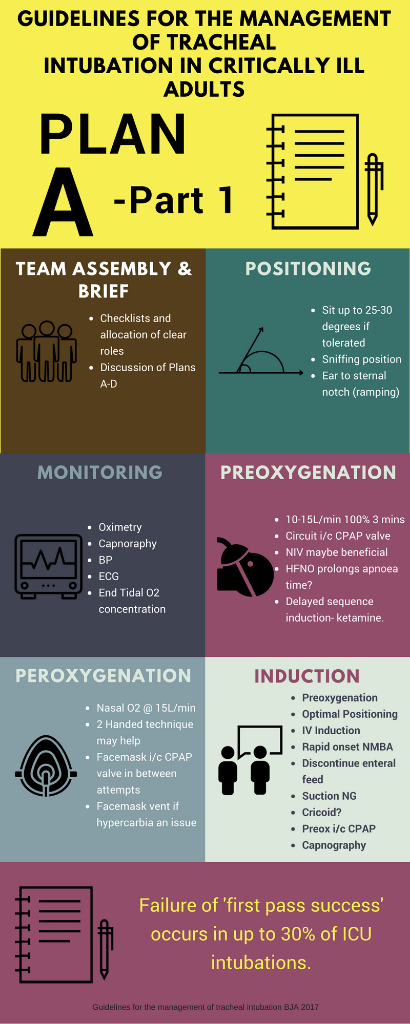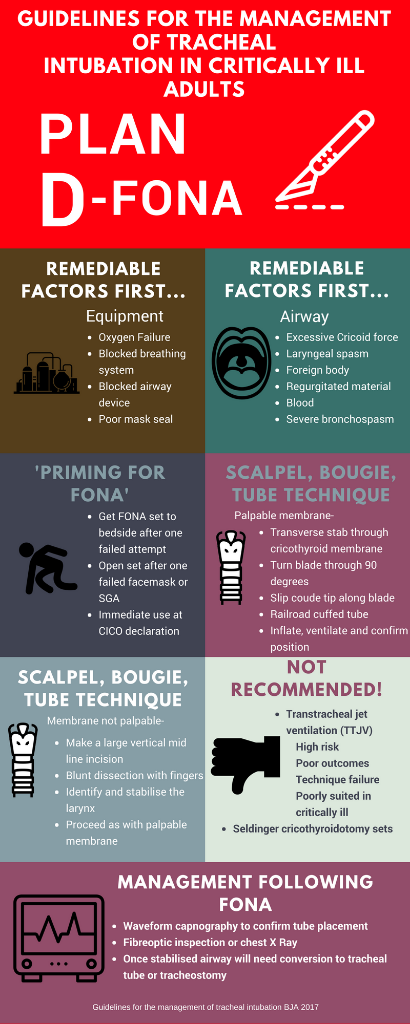## Mechanical Ventilation Series- A-a gradient.

### In order to understand the A-a gradient we need to first understand what affects the pressure of oxygen at four points in the respiratory system.

This includes the world around us from where we get our oxygen, so will also involve the atmosphere.

So lets break it down into the four A's:

Atmosphere

Airway

Alveoli

Artery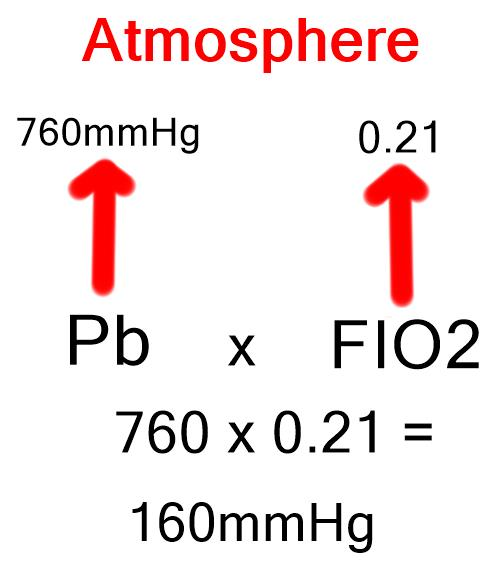To work out the pressure of oxygen in our atmosphere take the barometric pressure (760mmHg) at sea level, and multiply that by the concentration of oxygen in the atmosphere which is 21% (0.21).

### So the PO2 in the atmosphere is 160mmHg.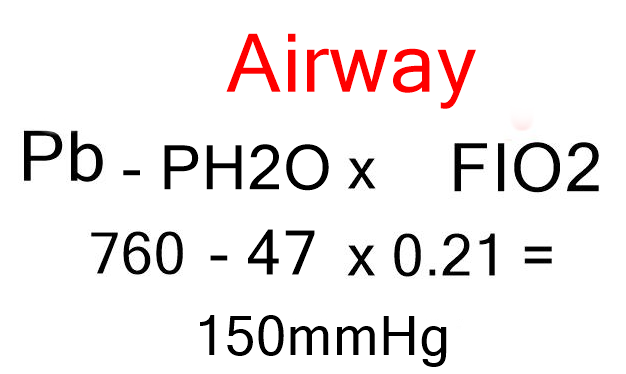As soon as this oxygen is inhaled it will lose pressure.

This is because there as water vapour which also exerts a pressure in the airways.

So now we have atmospheric pressure at sea level minus water vapour pressure multiplied by the percentage of oxygen.

### So the pressure of oxygen in the airway is now 150mmHg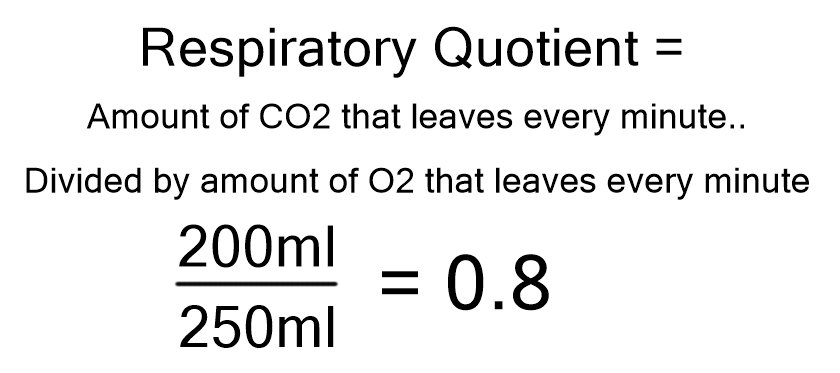The levels in the alveoli are further influenced by two other factors.

These are both related to the presence of CO2

This is leaving the body as the O2 is entering and the relationship between the two is known as the respiratory quotient.

### The respiratory quotient is 0.8

The pressure of CO2 in the alveoli is approximately 40mmHg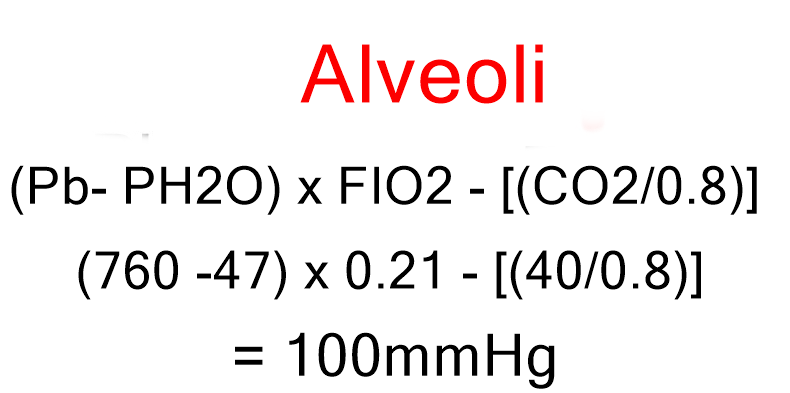So now to work out the pressure in the avleoli we need to take all the above into account.

We need to now subtract the partial pressure of CO2 (40mmHg) divided by the respiratory quotient.

### So the pressure of oxygen in the alveoli or the PAO2 is 100mmhg.

Note the capital A here.

## Artery

If there is no lung disease then the pressure of oxygen within the artery should also be 100mmHg.

### So the PaO2 should be 100mmHg

Note the small 'a' here.

## When calculating the gradient it is necessary to take into account the percentage of oxygen.

### Guidelines for the management of tracheal intubation in critically ill adults